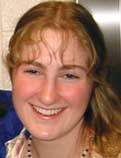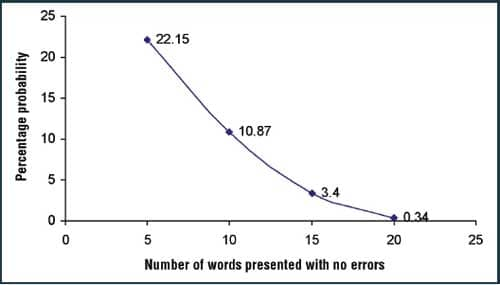Marshall Chasin, AuD, MSc, is an audiologist and director of auditory research at Musicians’ Clinics of Canada, Toronto. His daughter, Courtney Chasin, BASc, is a graduate student at the University of Calgary in Alberta.We have all had the problem of being behind schedule with patients and feeling the need to take short cuts to catch up. Some clinical compromises are just wrong, whereas others, if based on a sound rationale, can be useful. One question that frequently arises is whether hearing professionals need to present all 25 words in a word-recognition list if the patient gets the first 10 or so correct.

1. The “normal” range of scores on a word-recognition list is 88% to 100% (ie, up to three errors allowed in a 25-word list); and
2. The first 10 or 15 words presented are correct (ie, no errors made by the patient).

### One Man’s Poison Is Another’s Statistical Probability Calculation

Given these two assumptions, the following are two sets of calculations—one for stopping after 10 words, and the other for stopping after 15 words. For this analysis, the Poisson statistical distribution is used. The Poisson distribution is used whenever there is a “rare” event (such as an error in word recognition, or a car accident) and the number in the sample is relatively large (eg, 25 words).

An example of the use of this distribution is the case—albeit tragic—of audiology students getting hit by a car as they cross a busy street while rushing to class. If it is known that there is one accident each year, one can ask the probability of no students being hit each year, one student being hit each year, two students being hit each year, and so on. In this case, the “average” would be one accident/year.

Similarly, using this distribution, we can ask, “If we gave 10 words (of a 25-word list) and there were no errors in those first 10 words, what is the probability of getting more than three words wrong in the next 15 words of the list?” Three errors out of 25 words would yield a score of 88%, which is the lower limit of the normal range on word-recognition testing. To be exact, we must ask the related question, What is the probability of getting “no errors + one error + two errors + three errors in the next 15 words?” In the worst-case scenario, three errors would equate to 88% which is still in the normal range.

The Poisson distribution requires the calculation of the “average” of the rare event in order to determine the relevant probabilities.1 The distribution is shown by:

#### P(x) = e-avg (avg)x/x!

This looks complicated, but it is not. The letter e is the natural base, and e-avg can be found in tables in the back of statistics textbooks. P(x) is the calculated probability of x occurrences, or x errors, and x! is “x-factorial” (the multiple of numbers from 1 up to x). The “avg” is the average error rate, and is given by the number of words yet to be provided to the patient (ie, 15 in the case of only 10 words already given) multiplied by 0.12. This “avg” turns out to be 1.8. The figure of 0.12 is the difference of 100% minus 88% in decimals, assuming that 88% is the worst of what is considered the normal range. (If you wanted to recalculate the probabilities and assume that, for example, 90% was the minimum value for the normal range, the value would be 0.10 instead of 0.12.)

Specifically, we need to calculate:

• P(0) which is the probability that there are no errors in the next 15 words +
• P(1) which is the probability that there is one error in the next 15 words +
• P(2) which is the probability that there are two errors in the next 15 words +
• P(3) which is the probability that there are three errors in the next 15 words.

The sum of these four probabilities will add up to give a total probability of the chance that there will not be more than three errors in the next 15 words. In other words, we would have the estimate of a probability of a clinical error if we were to stop after only 10 words in a 25-word list, if no errors were found in the first 10 words.

The calculations are as follows: P(0) + P(1) + P(2) + P(3) = 16.53% + 29.75% + 26.78% + 16.07% = 89.13%. This means that, given the above assumption of no errors in the first 10 words, there is an 89.13% chance that the final word recognition score of the full 25-word list will not be less than 88%. Looking at it the other way, there is a 10.87% chance that the final score would be outside the normal range.

If we turn our attention to the question of using only the first 15 words of the 25- word list, assuming there are no errors up to that point, the calculations are similar, except that the “avg” term is now 10 words multiplied by 0.12, or 1.2. The answer to the calculation becomes: P(0) + P(1) + P(2) + P(3) = 30.11% + 36.14% + 21.68% + 8.67% = 96.60%. That is, if the first 15 words given in a word-recognition test are correct, there is only a 3.40% (100% to 96.60%) chance that there will be a final score of less than 88% correct.FIGURE 1. The estimated percent probabilities of being in error (ie, getting a final score of less than 88%) if one were to stop the test after only 5 words, 10 words, 15 words, and 20 words.

Figure 1 shows the estimated probability for the four cases of stopping after only five words presented with no errors, only 10 words with no errors, only 15 words with no errors, and only 20 words with no errors. Given that there are no word recognition errors, if stopping after 15 words—or 20 words—the estimated probabilities would be less than 5%.

It is, of course, a matter of clinical protocol whether such short cuts should be taken. These calculations are designed to provide an expected probability of being correct if the word list is terminated early, assuming correct responses for all the words already given. Acknowledging the above statistics, there are certainly other reasons for performing word-recognition scores in audiometry, such as performing an analysis (formal or informal) of the type and nature of the errors—useful information that can be used for counseling, as well as for aural rehabilitation.

The above calculations were performed for a 25-item word list with a gradually sloping performance-intensity function where the normal range has been set in the 88% to 100% range. Different calculations would need to be made for more steeply sloping word lists, such as the various speech-in-noise tests, and this is implicitly manifested in the range considered to be normal (eg, 88% to 100%).

It should be pointed out that these calculations are independent of the word list being used, and independent of hearing status. Implicit in the selection of the 88% to 100% range being accepted as “normal” is the nature of the word lists, the hearing status, and the slope of the performance-intensity function. It also becomes an entirely different statistical problem once errors are made, as this has been shown to be a binomial distribution (see Raffin and Schafer2).

### Conclusion

For those who wish to terminate a word list early if there are no errors, the calculated estimates of the probabilities of a final score outside the normal hearing range (<88%) is about 10.87% for stopping after 10 words, 3.40% for stopping after 15 words, and only 0.34% for stopping after 20 words.

None of the above should be taken as an endorsement of routinely cutting short your word-recognition testing; obviously, we owe our patients our best. However, if you are a busy hearing care clinician, you will doubtless run into situations where you are pressed for time and are tempted to cut short a word-list test. Now, at least, you’re aware of the odds.

### References

1. Pagano M, Gauvreau K. Principles of Biostatistics. 2nd ed. Pacific Grove, Calif: Thomson Learning; 2000.
2. Raffin MJM, Schafer D. Application of a probability model based on the binomial distribution to speech-discrimination scores. J Speech Hear Res. 1980; 23: 570-575.

Correspondence can be addressed to HR or Marshall Chasin, AuD, Musicians’ Clinics of Canada, #340 College Street, Toronto, Ontario, Canada, M5T 3A9; .